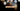# Leetcode | Solution of Valid Palindrome in JavaScript

March 28th, 2020
|

In this post, we will solve valid palindrome from leetcode and compute it's time and space complexities. Let's begin.

# Problem Statement

The question can be found at leetcode valid palindrome problem.

The problem states that we are given a string, and we need to check whether it is a palindrome or not.

# Constraints and challenges

The following constraints are given in the question

• We only need to consider alphanumeric characters (A-Z, a-z, 0-9) and ignore case
• An empty string is a valid palindrome

We'll see a solution using two pointers.

# Solution

We'll take the following approach

• First, convert the whole string to lower case (can convert to uppercase too)
• We'll place two pointers, one at the beginning and other at end of the string
• We'll check characters at both pointers.
• If the characters are alphanumeric, we'll check for the equality of the characters at both pointers

We have discussed the approach, I urge you to go ahead on leetcode and give it another try.If you are here, it means something went wrong in implementation or you are just too lazy. In any case, let's see a simple implementation of the above logic.
``````var isPalindrome = function (s) {
if (s.length === 0) return true;

s = s.toLowerCase();
let i = 0, j = s.length - 1;
while (i < j) {
if ((s[i] < 'a' || s[i] > 'z') && (s[i] < '0' || s[i] > '9')) {
i++;
continue;
}
if ((s[j] < 'a' || s[j] > 'z') && (s[j] < '0' || s[j] > '9')) {
j--;
continue;
}
if (s[i] !== s[j]) {
return false;
}
i++;
j--;
}

return true;
};``````

Let's look at the solution.

First, we handle the case of an empty string. Next, we convert the string to lower case. After that, we have taken two counters, one at position 0 other at the end of the string.

We are looping till both the pointers are the same, in which case it's already equal. Inside the loop, we check if the characters at the position are alphanumeric. In case they are not alphanumeric, we'll move the pointers inwards and continue with the loop. There's no point checking for palindrome at that index as the question ignores anything that is not alphanumeric.

In case both of the characters are alphanumeric, we check for their equality and return false if the characters are not equal.

Next, we update our pointers. Once we jump out of the loop, it means, every time the characterers were equal and the string is a palindrome, so we return true.

We can solve the question using a regex too but wanted to discuss a solution like this to explain that we can do logical operations on characters too.

Here are the stats

``````Status: Accepted
Runtime: 64ms
Memory: 34.7MB``````

## Time and space complexity

### Time complexity

We are looping over the string once in the worst case, so time complexity would be O(n).

### Space complexity

We are using to lower case function here and storing it back in the string. The operation would need a temporary space of length of the string. So space complexity is linear, O(n).

# Summary

So, we solved the valid palindrome problem by using two pointer method and calculated the time and space complexities.

I hope you enjoyed solving this question. This is it for this one, complete source code for this post can be found on my Github Repo. Will see you in the next one.